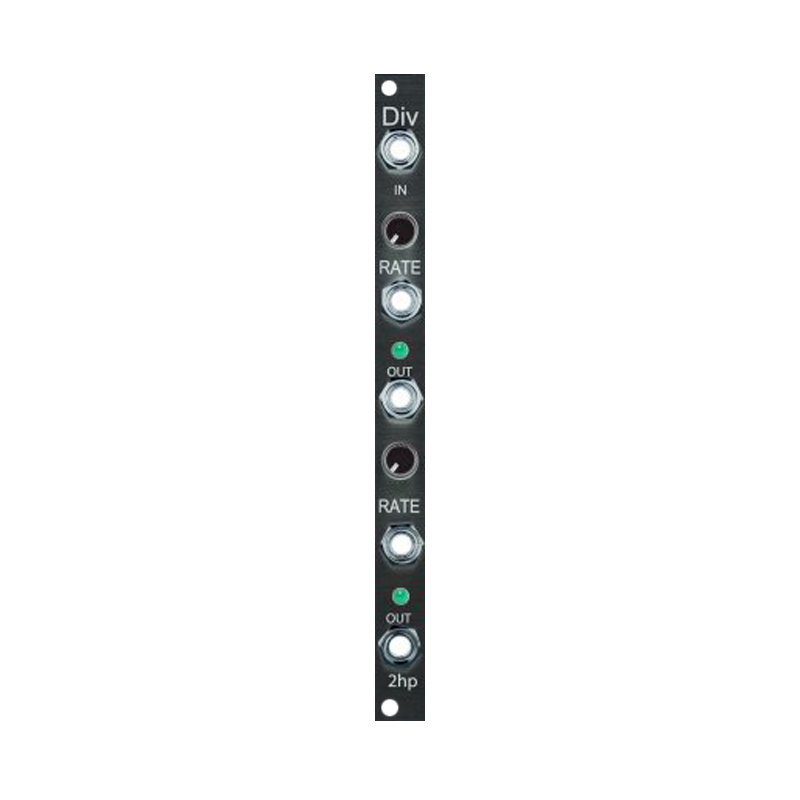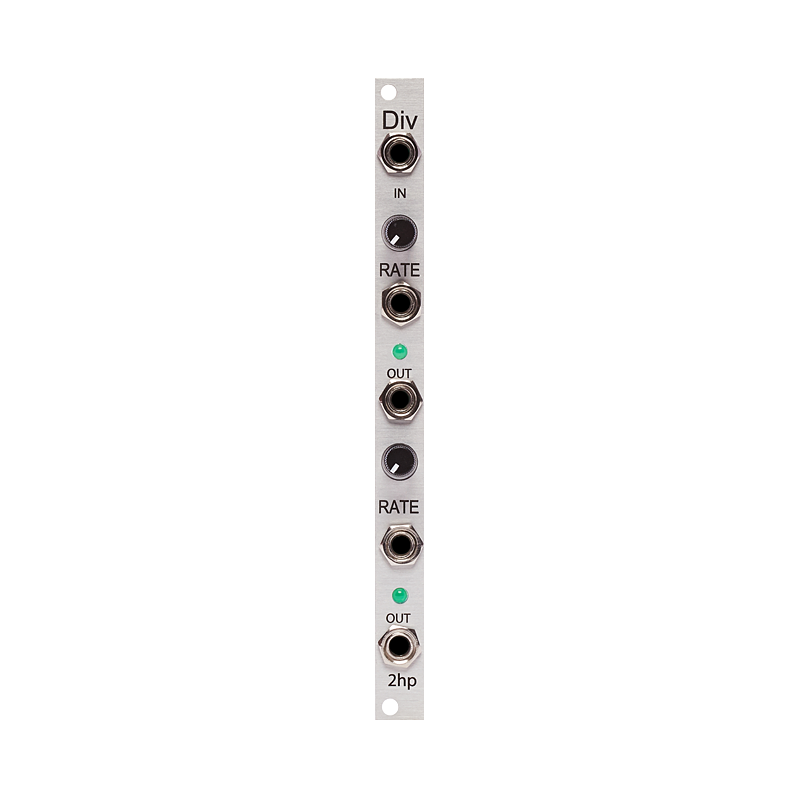# 2hp Div# 2hp Div

Write a review
Regular price
\$109.00
Sale price
\$109.00
Regular price

Color : Black
/ / /
Div is a 2 channel, voltage controlled clock divider and multiplier. Incoming clock signals can be multiplied or divided by a factor of 16 with a multitude of values in between. CV inputs provide external control of the current clock rate, allowing for the creation of dynamic rhythms from a single clock signal.
Div is a 2 channel, voltage controlled clock divider and multiplier. Incoming clock signals can be multiplied or divided by a factor of 16 with a multitude of values in between. CV inputs provide external control of the current clock rate, allowing for the creation of dynamic rhythms from a single clock signal.
Div is a 2 channel, voltage controlled clock divider and multiplier. Incoming clock signals can be multiplied or divided by a factor of 16 with a multitude of values in...

## Questions

Have any questions? We're here to help!